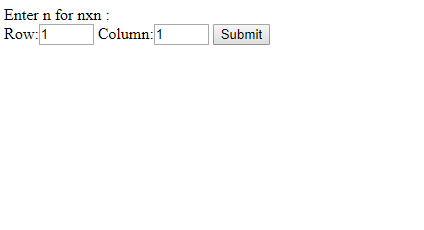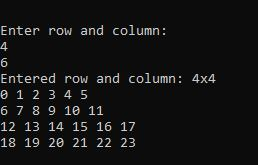# How to take user input for two dimensional (2D) array in PHP ?

There are two methods to take user input in PHP in two dimensional (2D) array.

Approach 1:

• Use HTML forms through PHP GET & POST method to take user input in two dimensional (2D) array.
• First, input data to HTML forms.
• Then use GET or POST method of PHP to get or post those input data into a variables.
• Finally, use those variable which holds input data and process using for loop.
• Though it is form two dimensional array so, you need two indices/variables for processing in for loop.
• Enter input one below another to state as two variables.

Example 1: Below example illustrate how to input user data for 2D-array using Form POST method.

 `"``; ` `echo` `"
` `    ``Row: ` `    ``Column: ` `    `` ` `"; ` ` `  `// Submit user iput data for 2D array ` `if` `(isset(``\$_POST``[``'submit'``])) { ` `     `  `    ``// POST submitted data ` `    ``\$dimention1` `= ``\$_POST``[``"1d"``]; ` `     `  `    ``// POST submitted data ` `    ``\$dimention2` `= ``\$_POST``[``"2d"``]; ` `     `  `    ``echo` `"Entered 2d nxn: "` `. ``\$dimention1` `            ``. ``"x"` `. ``\$dimention2` `. ``"
"``; ` `    ``\$d` `= []; ` `    ``\$k` `= 0; ` `     `  `    ``for``(``\$row` `= 0; ``\$row` `< ``\$dimention1``; ``\$row``++) { ` `        ``for` `(``\$col` `= 0; ``\$col` `< ``\$dimention2``; ``\$col``++) { ` `            ``\$d``[``\$row``][``\$col``]= ``\$k``++; ` `        ``} ` `    ``} ` `     `  `    ``for` `(``\$row` `= 0; ``\$row` `< ``\$dimention1``; ``\$row``++) { ` `        ``for` `(``\$col` `= 0; ``\$col` `< ``\$dimention2``; ``\$col``++) { ` `            ``echo` `\$d``[``\$row``][``\$col``].``" "``; ` `        ``} ` `        ``echo` `"
"``; ` `    ``} ` `} ` `?> `

Output:Approach 2:

• To take user input in php for two dimensional (2D) by using fopen() function which helps to get user input either at runtime or by external input file.
• First, assign those input data to variables.
• Finaly, use those variable which holds input data and process using for loop.
• Though it is form two dimensional array so, you need two indices/variables for processing in for loop.

Example: Below example illustrate how to input user data for 2D array using fopen() function.

 ` ` ` `

Output:My Personal Notes arrow_drop_upCheck out this Author's contributed articles.

If you like GeeksforGeeks and would like to contribute, you can also write an article using contribute.geeksforgeeks.org or mail your article to contribute@geeksforgeeks.org. See your article appearing on the GeeksforGeeks main page and help other Geeks.

Please Improve this article if you find anything incorrect by clicking on the "Improve Article" button below.